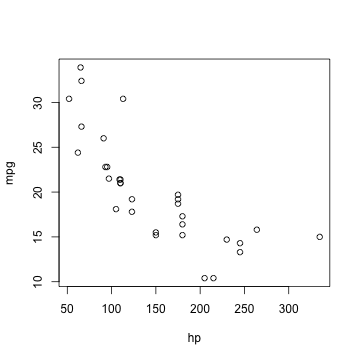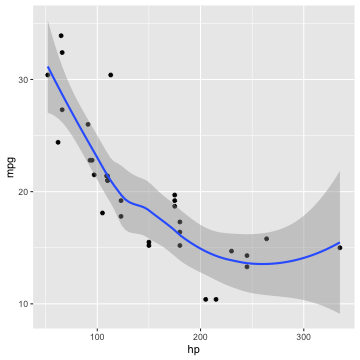```library(knitr)
opts_chunk\$set(fig.width = 5, fig.height = 5)
```

This is a minimal example which shows knitr working with HTML pages. See here for the output and here for the source.

Boring stuff as usual:

```## a simple calculator
1 + 1
```
```##  2
```
```## boring random numbers
set.seed(123)
rnorm(5)
```
```##  -0.56048 -0.23018  1.55871  0.07051  0.12929
```

We can also produce plots (centered by the option `fig.align='center'`):

```library(ggplot2)
plot(mpg ~ hp, mtcars)
``````ggplot(mtcars, aes(hp, mpg)) + geom_smooth()
```Errors, messages and warnings can be put into `div`'s with different `class`es:

```sqrt(-1)  # warning
```
```## Warning in sqrt(-1): NaNs produced
```
```##  NaN
```
```message("knitr says hello to HTML!")
```
```## knitr says hello to HTML!
```
```1 + "a"  # mission impossible
```
```## Error in 1 + "a": non-numeric argument to binary operator
```

In the end, let's show off a 3D plot from the rgl package.

```library(rgl)
knit_hooks\$set(rgl = hook_rgl)  # set up the hook first
```
```plot3d(data.frame(x = rnorm(100), y = rnorm(100), z = rnorm(100)), col = rainbow(100))
par3d(zoom = 0.8)
```Well, everything seems to be working. Let's ask R what is the value of π? Of course it is `3.1416`.